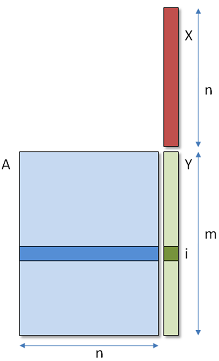# GPU matrix-vector product (gemv)

Eric Bainville - Feb 2010

## One thread per dot product

In this first implementation, we will run m threads, and each thread will compute one coefficient of Y.One thread produces one component i of the result, by computing the dot product between row i of the matrix (blue) and vector X (red).
```__kernel void gemv1(__global const scalar_t * a,__global const scalar_t * x,
__global scalar_t * y,int m,int n)
{
scalar_t sum = 0.0f;
int i = get_global_id(0); // row index
for (int k=0;k<n;k++)
{
sum += a[i + m*k] * x[k];
}
y[i] = sum;
}
```

The best timings for the work group size WG between 1 and the max allowed value are:

```GTX285, v1, float:  M = 31 GB/s   C = 15 GFlops   (WG=64)
HD5870, v1, float:  M =  1.4 GB/s C =  0.7 GFlops (WG=64)

GTX285, v1, double: M = 57 GB/s   C = 14 GFlops   (WG=32)
HD5870, v1, double: M =  1.5 GB/s C =  0.4 GFlops (WG=64)
```

Here, each entry of A is read only one time, but each component of X is read m times from global memory. Despite of this, the memory throughput is not so bad for the GTX285, because all threads executing simultaneously (i.e. in one warp) use the same value Xk, and benefit from a single memory access broadcast to all threads. The HD5870 does not benefit yet from the access broadcast.

In the next page, instead of one thread per dot product, the computation will be carried out by p concurrent threads.